Computers, Materials & ContinuaDOI:10.32604/cmc.2021.016770Article

An Adaptive Lasso Grey Model for Regional FDI Statistics Prediction

1Centre for Innovation Research in Social Governance, Changsha University of Science and Technology, Changsha, 410114, China
2College of Information Technology and Management, Hunan University of Finance and Economics, Changsha, 410205, China
3Department of Mathematics and Computer Science, Northeastern State University, Tahlequah, 74464, USA
*Corresponding Author: Huajun Huang. Email: huanghuajun@hufe.edu.cn
Received: 11 January 2021; Accepted: 26 April 2021

Abstract: To overcome the deficiency of traditional mathematical statistics methods, an adaptive Lasso grey model algorithm for regional FDI (foreign direct investment) prediction is proposed in this paper, and its validity is analyzed. Firstly, the characteristics of the FDI data in six provinces of Central China are generalized, and the mixture model's constituent variables of the Lasso grey problem as well as the grey model are defined. Next, based on the influencing factors of regional FDI statistics (mean values of regional FDI and median values of regional FDI), an adaptive Lasso grey model algorithm for regional FDI was established. Then, an application test in Central China is taken as a case study to illustrate the feasibility of the adaptive Lasso grey model algorithm in regional FDI prediction. We also select RMSE (root mean square error) and MAE (mean absolute error) to demonstrate the convergence and the validity of the algorithm. Finally, we train this proposedal gorithm according to the regional FDI statistical data in six provinces in Central China from 2006 to 2018. We then use it to predict the regional FDI statistical data from 2019 to 2023 and show its changing tendency. The extended work for the adaptive Lasso grey model algorithm and its procedure to other regional economic fields is also discussed.

Keywords: Adaptive lasso grey model algorithm; regional FDI statistics; mean value of regional FDI; median value of regional FDI

1  Introduction

Economic development varies from country to country, and the influencing factors of the regional FDI are also varied . The regional FDI statistics can accurately and effectively describe the relationship among basic situation, influencing factors and investment trend of regional FDI.

How to effectively predict regional FDI statistics to improve the regional economy is a complicated problem. In the past decade, many traditional statistical methods  have been proposed to solve this problem. Being empirical or semi-empirical, these models can provide neither specific assumptions nor sufficent statistical data.

Lasso's method can effectively overcome the above deficiency of the traditional statistical methods. In this method,proper variables with a significant impact can be selected to reduce the complexity of data  and display the influence of all variables on the estimated parameters . However, Lasso’s method has some defects in precision. The adaptive Lasso method  assigns different weights to different coefficients to improve the accuracy of calculation parameters.

In recent years, many studies have shown that the grey theory is a valid method that can correctly predict the properties in some fields  by mining some available information and extracting valuable key information. The regional FDI system is a typical grey system suitable for the grey model with both the evident hierarchy complexity and the constant change, and its index characteristic data is uncertain and incomplete . Therefore, it is feasible to combine the adaptive Lasso method and the grey model, i.e., to establish an adaptive Lasso grey algorithm to predict regional FDI statistics.

2  Adaptive Lasso Grey Model Algorithms Predicting Regional FDI Statistics

Many methods [22,23] have been proposed to solve the Lasso problem. However, these methods can only deal with big data, not minor data problems. Therefore, the adaptive Lasso model  and the grey model  are needed to precisely calculate the predicted value. Based on characteristics [30,31] and the regional FDI statistics variables, the main algorithm in this paper is described as follows.

According to the data of regional FDI, let: x1,x2,,xp represents the factors, and n denotes the sample number. The sample matrix can be described as X=[x11x12x1px21x22x2pxn1xn2xnp], where xj=[x1jx2jxnj]. So, FDI statistics are represented as y=[y1y2yn] andβ=[β1β2βp].

Adaptive Lasso Grey Model Algorithm Predicting Regional FDI Statistics:

Step 1: Investigate the possible factors of regional FDI and obtain their specific data.

Step 2: Set the value range of the variables sample matrix {x1,x2,,xp} and the learning top limit T.

Step 3: Specify the required statistics (the regional FDI data, the mean and median values influencing factors) and get the statistical matrix X.

Step 4: Initialize β and solve the least-squares estimation y = Xβ, then get β.

Step 5: Compute the weight vector:

ωj^=1βj(j=1,2,,p). (1)

Step 6: For the adaptive Lasso model: minβ{yXβ2+λj=1pωj^|βj|}, set xj=xjωj^ (j=1,2,,p), and establish the substituted model:

minβ{yj=1pxj(ωj^βj)2+λj=1pωj^|βj|}. (2)

Step 7: Set β=ω^β, f(β)=yj=1pxjβ2 and g(β)=j=1p|βj|=β1, where βk is the kth result of β, then compute βk+1:

βk+1=minβ{f(β)+λg(β)}=minβ{L2βz22+λβ1}, (3)

where z=βk1Lf(βk), and L is a constant.

Step 8: Let F(β)=L2j=1p(βjzj)2+λj=1p|βj|. For βj (the jth result of β), obtain the optimal values: βj: F(x)βj=0, then compute:

βj=sgn(zj)max(|zj|λL,0). (4)

Step 9: If |(f(βk+1)+λg(βk+1))(f(βk)+λg(βk))|<104 or the learning number reaches T, end the algorithm, otherwise jump to Step 4.

Step 10: Establish the adaptive Lasso model according to βj^=βωj^, j=1,2,,p.

Step 11: Select x, get xj=[x1j,x2j,,xnj]T, set φi(0)=xij, i=1,2,,n, and compute φ(1)=(φ1(1),φ2(1),,φn(1)), where βj^ is non-zero, and

φr(1)=i=1rφi(0)=φr1(1)+φr(0),r=1,2,,n. (5)

Step 12: Substitute φ(1) into the grey model dφ(1)dt+aφ(1)=bφ=Bϕ, where φ=[φ2(0)φ3(0)φn(0)], B=[12[φ2(1)+φ1(1)]112[φ3(1)+φ2(1)]112[φn(1)+φn1(1)]1], and ϕ=[ab]. Compute ϕ through

[ab]=[a^b^]=(BTB)1BTφ. (6)

Step 13: Solve dφ(1)dt+aφ(1)=b, get φt(1)=(φ1(1)ba)ea(t1)+ba, set r=t1, compute φr+1(1)=(φ1(1)ba)ear+ba and restore φr+1(1) to φr+1(0) after accumulation:

φr+1(0)=φr+1(1)φr(1)=(ea1)(φ1(1)ba)ear(r=1,2,,). (7)

Step 14: Substitute (6) into (7), get:

φr+1(0)=(ea^1)(φ1(1)b^a^)ea^r. (8)

if r=1,2,,n1, compute the fitted value φr+1(0), or compute the predicted value φr+1(0).

Step 15: For all βj^ non-zero factors xj with non-zero βj^, repeat Steps 11–14, predict the values of xj (j=1,2,,p) in the next predicting years.

Step 16:Establish the adaptive Lassogrey model (Step10) and compute the regional FDI statistics y for future years.

3  A Case Study

Among many forms of regional FDI statistics,this paper only considers the mean and median values to illustrate the feasibility and effectiveness of our proposed algorithm.. Taking six provinces of Central China as the case for study, through numerical analysis of regional FDI, their overall regional FDI capacity is judged , which provides reference for formulating related policies.

In this case study , we select the data of regional FDI from 2006 to 2018, such as the annual regional GDP (x1), the average wages (x2), the total investment value in fixed assets (x3), the highway mileage (x4), the total import and export trade value (x5), the ratio of the industrial added value increment (x6), the expenditure of government personnel (x7), the total freight (x8), the total retail sales of the consumer goods (x9), the number of patents (x10), the proportion of the fiscal expenditure in GDP (x11), the number of the designated size industries (x12), the number of students in higher education (x13) and the amount of FDI inflows in the previous five years (x14), etc. The mean and median values of the above 14 factors were taken as the input data of the algorithm. To verify the feasibility and effectiveness of the algorithm, 80% of the samples are randomly selected as training samples, and the remaining 20% as testing samples. The natural logarithm of the time series data is processed to eliminate various characteristics on the data. Note that we only select the mean and median values shown in Tabs. 1 and 2, respectively, due to the limited space. For specific data, please refer to the China Statistical Searbook and the Provincial Statistical Yearbooks in China.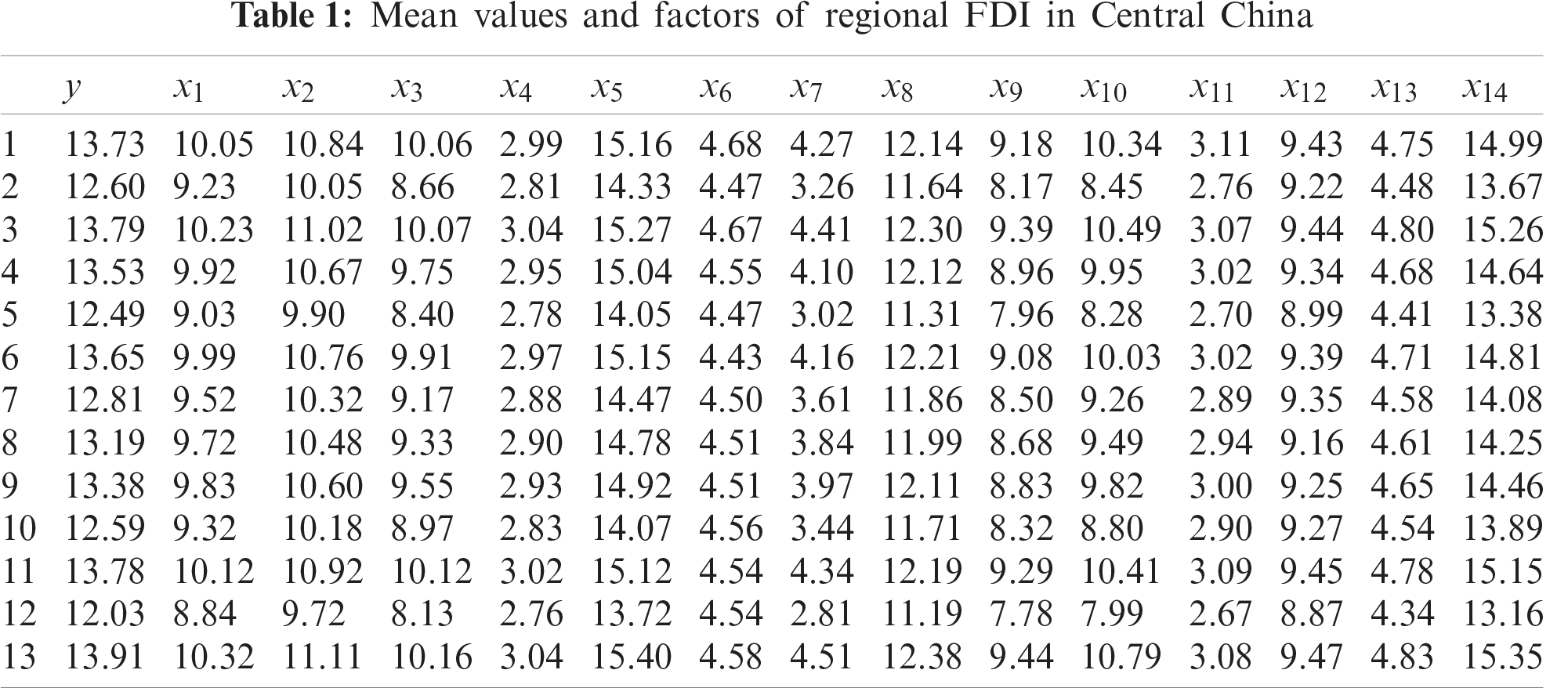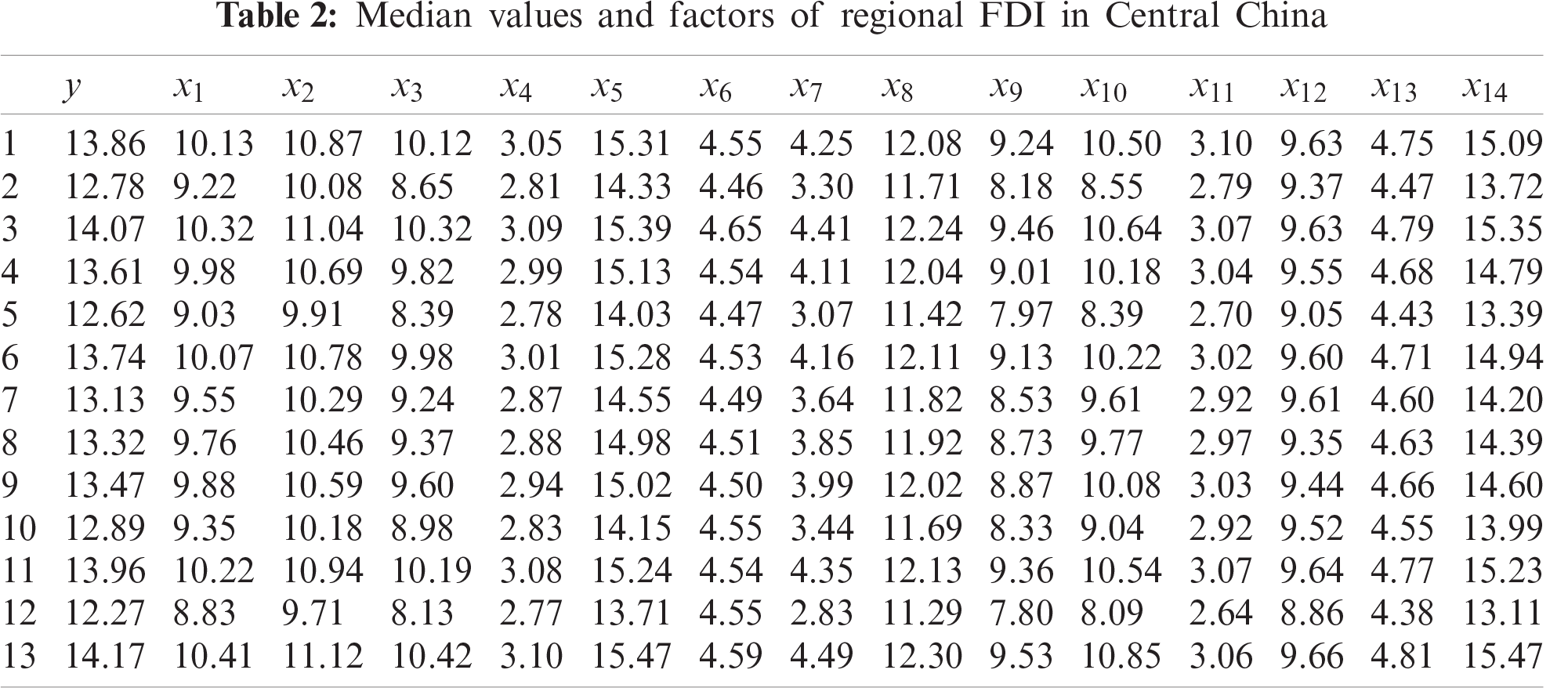By the above algorithm of the adaptive Lasso, the estimated coefficients of the specific data for regional FDI in Central China are computed and outlined in Tab. 3.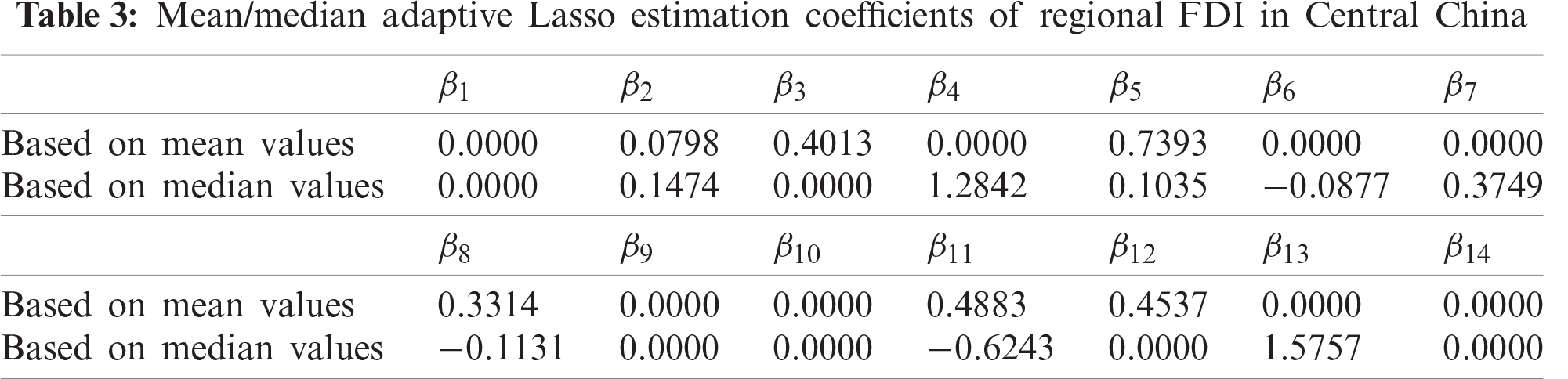It can be seen from the second line in Tab. 3 that x1, x4, x6, x7, x9, x10, x13 and x14 are eliminated because their coefficients are all 0 according to the algorithm used to calculate the mean value of regional FDI. Similarly from the third line, x1, x3, x9, x10, x12 and x14 are independent of the median value of regional FDI. Also, the mean and their impact intensity are different from those of the median. The algorithm can eliminate variables and has unique advantages in the case of multiple indicators.

In order to verify the effectiveness and rationality of the adaptive Lasso grey model algorithm,RMSE (the root mean square error)  and MAE (the mean absolute error) are selected to evaluate it. Set h(xi), (i=1,2,,n) as the computed results and yi, (i=1,2,,n) as the actual values, RMSE and MAE can be represented as follows:

RMSE=i=1n(h(xi)yi)2n, (9)

MAE=1ni=1n|h(xi)yi|. (10)

RMSE and MAE can be computed by this algorithm, and the results are shown in Tab. 4. It can be found that RMSE and MAE are relatively small, indicating that the selected variables can well reflect the factors related to the regional FDI statistics.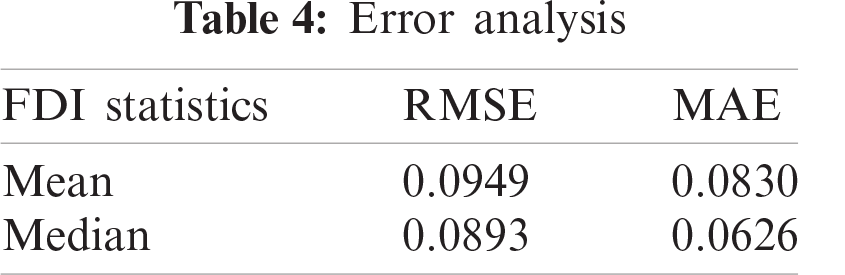Based on the coefficients in Tab. 3, we select six primary factors affecting the mean value of FDI and eight main factors affecting the median value of FDI, and used the remaining part of the algorithm to predict the factors affecting regional FDI statistics from 2019 to 2023. The prediction accuracy is shown in Tabs. 5 and 6. The predicted and actual values of the affecting variables are obtained through Python and plotted in Figs. 1 and 2.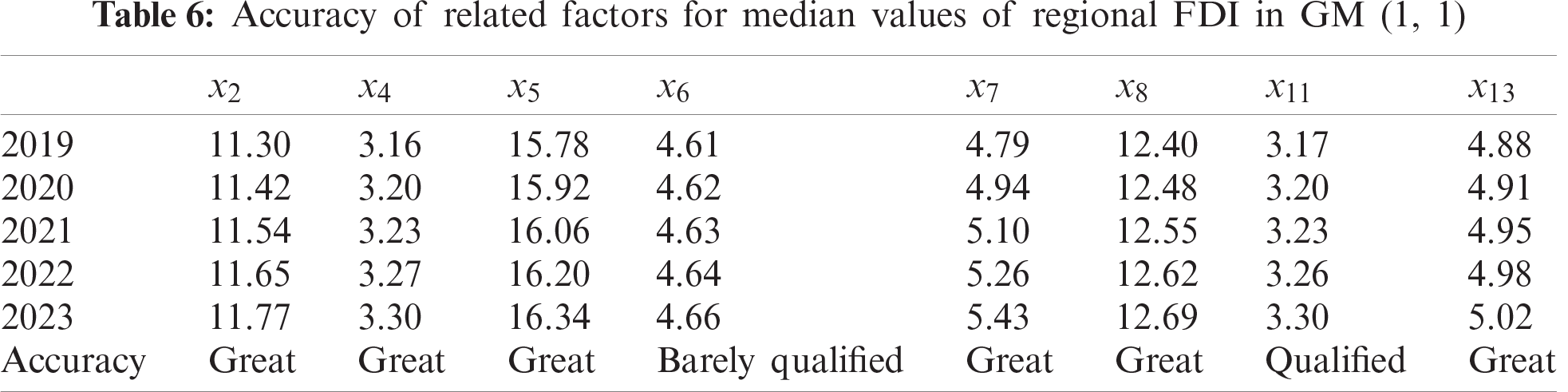Figure 1: Predicted and actual factor values of mean regional FDI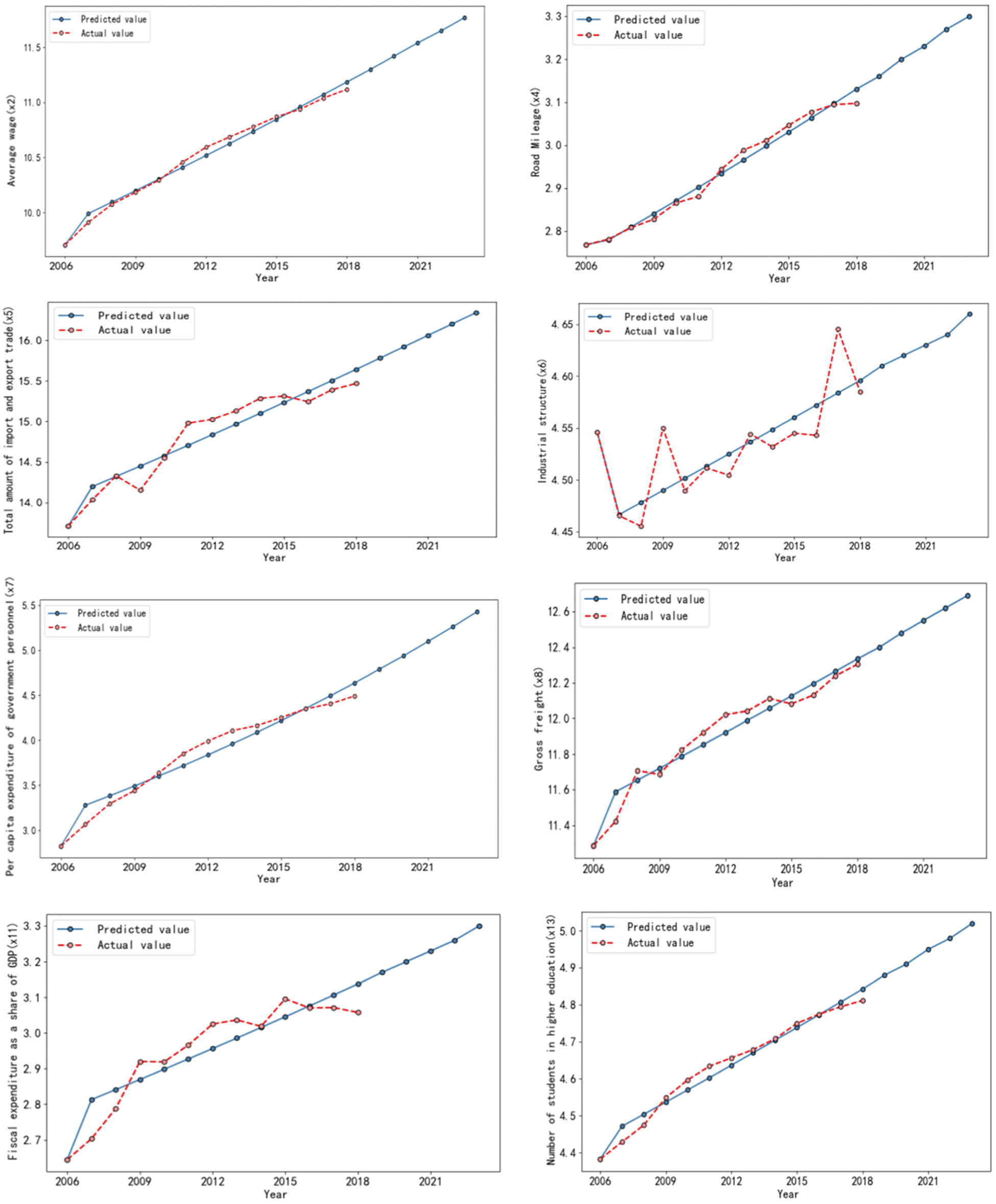Figure 2: Predicted and actual factor values of median regional FDI

It can be seen from Figs. 1 and 2 that the predicted factor values of regional FDI statistics are close to the actual factor values, which indicates that what is predicted is valid. Moreover, Tabs. 5 and 6 demonstrate that these explanatory variables have many advantages, and various regional FDI statistics have different affecting factors. Considering the computed results and error analysis, the prediction accuracy of this algorithm is gererally satisfying, and the grey GM (1, 1) model combined with the adaptive Lasso model has a good effect on short-term single-factor prediction.

Using the adaptive Lasso grey model algorithm, the statistical data of regional FDI in six provinces of Central China from 2006 to 2023 were predicted . The comparison between the predictedand actual values of regional FDI is shown in Fig. 3.Figure 3: Predicted and actual values of regional FDI statistics in Central China

Fig. 3 shows that the predicted values from 2006 to 2018 are very close to the actual value, and demonstrates that the adaptive Lasso grey model algorithm is valid in regional FDI statistics. It should be noted that no correlational FDI value could be forecasted with the fast change of the main factors of FDI statistics, the reasons of which is the focus of our future work.

4  Conclusions

By optimizing some traditional mathematical statistical methods, this paper proposes an adaptive Lasso grey model to predict regional FDI statistics. Based upon the characteristics of FDI data of six provinces of Central China, a test was designed to verify the effect of this adaptive Lasso grey model. Meanwhile, the feasibility and validity of the main algorithm of regional FDI statistics are demonstrated. This study also shows that the adaptive Lasso grey model with its algorithm and procedure can be extended to regional GDP and income study..

Acknowledgement: The author would like to thank the equipment support of Changsha University of Science and Technology as well as the support of the Fund Project.

Funding Statement: This work was supported in part by the National Key R&D Program of China (No. 2019YFE0122600), author H. H, https://service.most.gov.cn/; in part by the Project of Centre for Innovation Research in Social Governance of Changsha University of Science and Technology (No. 2017ZXB07), author J. H, https://www.csust.edu.cn/mksxy/yjjd/shzlcxyjzx.htm; in part by the Public Relations Project of Philosophy and Social Science Research Project of the Ministry of Education (No. 17JZD022), author J. L, http://www.moe.gov.cn/; in part by the Key Scientific Research Projects of Hunan Provincial Department of Education (No. 19A015), author J. L, http://jyt.hunan.gov.cn/; and in part by the Hunan 13th five-year Education Planning Project (No. XJK19CGD011), author J. H, http://ghkt.hntky.com/.

Conflicts of Interest: The authors declare that they have no conflicts of interest to report regarding the present study.

References

1.  K. Kirkham, “The formation of the Eurasian economic union: How successful is the Russian regional hegemony,” Journal of Eurasian Studies, vol. 7, no. 2, pp. 111–128, 2016.

2.  T. Ait-Izem, M. Harkat, M. Djeghaba and F. Kratz, “On the application of interval PCA to process monitoring: A robust strategy for sensor FDI with new efficient control statistics,” Journal of Process Control, vol. 63, pp. 29–46, 2018.

3.  J. R. Afonso, E. C. Araújo and B. G. Fajardo, “The role of fiscal and monetary policies in the Brazilian economy: Understanding recent institutional reforms and economic changes,” The Quarterly Review of Economics and Finance, vol. 62, pp. 41–55, 2016.

4.  X. H. Zhao, L. Niu, C. Clerici, R. Russo, M. Byrd et al., “Dataanalysisof MS-based clinical lipidomics studies with crossover design: A tutorial mini-review of statistical methods,” Clinical Mass Spectrometry, vol. 13, pp. 5–17, 2019.

5.  T. Kishi, Y. Matsuda, K. Sakuma, M. Okuya and N. Iwata, “Factors associated with discontinuation in the drug and placebo groups of trials of second-generation antipsychotics for acute schizophrenia: A meta-regression analysis: Discontinuation in antipsychotic trials,” Journal of Psychiatric Research, vol. 130, pp. 240–246, 2020.

6.  Y. Y. Zou, G. L. Fan and R. Q. Zhang, “Quantile regression and variable selection for partially linear single-index models with missing censoring indicators,” Journal of Statistical Planning and Inference, vol. 204, pp. 80–95, 2020.

7.  Z. C. Chen, Y. Q. Bao, H. Li and F. B., “SpencerLQD-RKHS-based distribution-to-distribution regression methodology for restoring theprobabilitydistributionsof missing SHM data,” Mechanical Systems and Signal Processing, vol. 121, pp. 655–674, 2019.

8.  A. Katrutsa and V. Strijov, “Comprehensive study of feature selection methods to solve multicollinearity problem according to evaluation criteria,” Expert Systems with Applications, vol. 76, pp. 1–11, 2017.

9.  C. Chang and R. S. Tsay, “Estimation of covariance matrix via the sparse Cholesky factor with lasso,” Journal of Statistical Planning and Inference, vol. 140, no. 12, pp. 3858–3873, 2010.

10. E. Lindström and J. Höök, “Unbiased adaptive lasso parameter estimation for diffusion processes,” IFAC-PapersOnLine, vol. 51, no. 15, pp. 257–262, 2018.

11. B. Zeng, H. M. Duan, Y. Bai and W. Meng, “Forecasting the output of shale gas in China using an unbiased grey model and weakening buffer operator,” Energy, vol. 151, pp. 238–249, 2018.

12. X. P. Xiao, H. M. Duan and J. H. Wen, “A novel car-following inertiagraymodeland its application in forecasting short-term traffic flow,” Applied Mathematical Modelling, vol. 87, pp. 546–570, 2020.

13. R. Dash, “An improved shuffled frog leaping algorithm based evolutionary framework for currency exchange rate prediction,” Physica A: Statistical Mechanics and its Applications, vol. 486, no. 16, pp. 782–796, 2017.

14. Q. Liu, X. Y. Xiang, J. H. Qin, Y. Tan, J. S. Tan et al., “Coverless steganography based on image retrieval of DenseNet features and DWT sequence mapping,” Knowledge-Based Systems, vol. 192, pp. 105375–105389, 2020.

15. Y. J. Luo, J. H. Qin, X. Y. Xiang and Y. Tan, “Coverless image steganography based on multi-object recognition,” IEEE Transactions on Circuits and Systems for Video Technology, 2021. https://doi.org/10.1109/TCSVT.2020.3033945.

16. J. H. Qin, J. Wang, Y. Tan, H. J. Huang, X. Y. Xiang et al., “Coverless image steganography based on generative adversarial network,” Mathematics, vol. 8, no. 1394, pp. 1–11, 2020.

17. W. T. Ma, J. H. Qin, X. Y. Xiang, Y. Tan and Z. B. He, “Searchable encrypted image retrieval based on multi-feature adaptive late-fusion,” Mathematics, vol. 8, no. 1019, pp. 1–15, 2020.

18. Z. D. Wang, J. H. Qin, X. Y. Xiang and Y. Tan, “A privacy-preserving and traitor tracking content-based image retrieval scheme in cloud computing,” Multimedia Systems, 2021. https://doi.org/10.1007/s00530-020-00734-w.

19. J. H. Qin, W. Y. Pan, Y. Tan, X. Y. Xiang and G. M. Hou, “A biological image classification method based on improved CNN,” Ecological Informatics, vol. 58, pp. 1–8, 2020.

20. Z. Zhou, J. H. Qin, X. Y. Xiang, Y. Tan, Q. Liu et al., “News text topic clustering optimized method based on IF-IDF algorithm on spark,” Computers, Materials & Continua, vol. 62, no. 1, pp. 217–231, 2020.

21. T. Xu, M. Zhao, X. Yao and K. He, “An adjust duty cycle method for optimized congestion avoidance and reducing delay for wsns,” Computers, Materials & Continua, vol. 65, no. 2, pp. 1605–1624, 2020.

22. R. Tibshirani, “Regression shrinkage and selection via the lasso,” Journal of the Royal Statistical Society, vol. 15, no. 1, pp. 267–288, 1996.

23. B. Efron, T. Hastie, I. Johnstone and R. Tibshirani, Least Angle Regression. New York: Springer, 1999.

24. H. Zou, “The adaptive lasso and its oracle properties,” Journal of the American Statistical Association, vol. 101, no. 476, pp. 1418–1429, 2006.

25. W. P. Wang and J. L. Deng, “Study on chaotic characteristics of GM (1,1) model in grey system,” Systems Engineering, vol. 6, no. 2, pp. 13–16, 1997.

26. J. Z.Chen, “GM (1,1) model and curve AeTx fitting,” Systems Engineering, vol. 8, no. 4, pp. 67–71, 1988.

27. P. R. Ji, X. Y. Hu and D. Q. Xiong, “Analysis and evaluation of grey prediction model,” Hydroelectric Energy Science, vol. 17, no. 2, pp. 42–44, 1999.

28. Y. Mu, “Direct modeling method of unbiased grey GM (1,1) model,” Systems Engineering, vol. 25, no. 9, pp. 1094–1107, 2003.

29. Z. X. Wang, Y. G. Dang and S. F. Liu, “Analysis of chaotic characteristics of unbiased GM (1,1) model,” Systems Engineering, vol. 11, pp. 153–158, 2007.

30. Y. T. Chen, L. W. Liu, V. Phonevilay, K. Gu, R. L. Xia et al., “Image super-resolution reconstruction based on feature map attention mechanism,” Applied Intelligence, 2021. https://doi.org/10.1007/s10489-020-02116-1.

31. Y. T. Chen, L. W. Liu, J. J. Tao, X. Chen, R. L. Xia et al., “The image annotation algorithm using convolutional features from intermediate layer of deep learning,” Multimedia Tools and Applications, vol. 80, no. 3, pp. 4237–4261, 2020.

32. X. W. Liang, Y. L. Luo and D. Y. Peng, “Comprehensive evaluation of industrial carrying capacity in Central China,” Finance and Economics, vol. 2020, no. 7, pp. 91–96, 2020.This work is licensed under a Creative Commons Attribution 4.0 International License, which permits unrestricted use, distribution, and reproduction in any medium, provided the original work is properly cited.